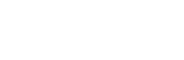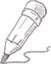#liting李

2021-04-23   阅读量: 25

Python

## Python如何将字典（dict）转化为Dataframe？

>>> dict = {'a':'apple','b':'banana'}

>>> dict

{'a': 'apple', 'b': 'banana'}

>>> import pandas as pd

>>> df = pd.DataFrame([dict])

>>> df

a b

0 apple banana

1、 使用DataFrame的from_dict()方法。

>>> df = pd.DataFrame.from_dict(dict, orient='index',columns=['fruits'])

>>> df = df.reset_index().rename(columns = {'index':'id'})

>>> df

id fruits

0 a apple

1 b banana

>>>

2、将字典转变为Series，再转为DataFrame。

>>> df = pd.DataFrame(pd.Series(dict), columns=['fruits'])

>>> df = df.reset_index().rename(columns={'index':'id'})

>>> df

id fruits

0 a apple

1 b banana

>>>

>>> df = pd.DataFrame([dict]).T

>>> df = df.reset_index().rename(columns={'index':'id'})

>>> df

id 0

0 a apple

1 b banana

92.3077 1 0 关注作者 收藏

## 评论(0)